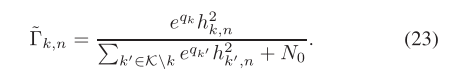# Q Cannot perform the operation: {real affine} ./ {real affine}

How to solve this problem in CVX：
`trace(hb_s(:,a)*hb_T(a,:)*T)/hb_s(:,b)*hb_T(b,:)*T+sigma_e^2>=1000`

both trace(hb_s(:,a)*hb_T(a,:)*T) and hb_s(:,b)*hb_T(b,:)*T+sigma_e^2 are complex

Disciplined convex programming error:
Cannot perform the operation: {real affine} ./ {real affine}

You have not shown us enough to reconcile what you say with the error message. if it is really `{real affine} ./ {real affine}` and the denominator is positive, then you could multiply all the terms by the denominator and (presumably) get a real affine inequality. If that is not the case, then please show us a complete reproducible problem, Your inequality wouldn’t appear to make sense if the LHS does not evaluate to real.

Thank for your advice. The problem has been solvedvariables q(K,1)
expressions tau_kn(K,N);
tau_kn=1./q(1)

Disciplined convex programming error:
Cannot perform the operation: {positive constant} ./ {real affine}

This is an English language forum.

Use `tau_kn=inv_pos(q(1));` . please see [http://cvxr.com/cvx/doc/](CVX Users’ Guide), for the functions and DCP rules in there.

Note that use of `inv_pos` effectively constrains its argument, q(1)` to be nonnegative

Dear Mark
I input this “tau_kn=q(1).*h_kn(1,1).^2.*q(2)” ,it doesn’t have any problems(q is variable, h_kn is known),but I input “tau_kn=q(1).*h_kn(1,1).^2.inv_pos(q(2)) ",error is "Cannot perform the operation: {real affine} .{convex}” could you tell me any advices?

Dear Jack
I input this “tau_kn=q(1).*h_kn(1,1).^2.*q(2)” ,it doesn’t have any problems(q is variable, h_kn is known),but I input “tau_kn=q(1).*h_kn(1,1).^2.inv_pos(q(2)) ",error is "Cannot perform the operation: {real affine} . {convex}” could you tell me any advices?

This is not a normal error but a DCP error. To know why, you have to learn DCP ruleset in the link above. Basically, CVX only accepts combinations of certain convex functions by certain rules.

Please show a minimum reproducible problem, so that it is clear exactly what the variables are. If `q` is a variable, I don’t see why even the 1st form would be accepted, because it involves the product of variables ( `q(1)` and `q(2)` ).

In this problem, Q and X are variables. This problem uses the Q value obtained in the last iteration as part of the parameters. In CVX, the variable is not allowed to set the initial value, so in the first iteration, I said that the Q value and the value of the previous iteration are set as variables. Then the program is a non convex problem, and the program will report an error. How should I solve this convex optimization problem that requires the value of the last iteration.The distinction between MATLAB variable and CVX variable is crucial. The optimal value from the previous iteration would be a MATLAB variable, containing a double precision numerical value, and should not be a CVX variable or expression. Y

You need to set an initial value for this MATLAB variable prior to the first cvx_begin. Do not declare it as a CVX variable if that would make the optimization problem non-convex.

I see it. I’ll try it now. Thank you!!